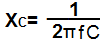﻿ Capacitor Impedance Calculator ﻿# Capacitor Impedance CalculatorThe capacitance impedance calculator calculates the impedance of a capacitor based on the value of the capacitance, C, of the capacitor and the frequency, f, of the signal passing through the capacitor, according to the formula, XC=1/(2πfC).

A user enters the capacitance, C, and the frequency, f, and the result will automatically be calculated and shown. The impedance result which is displayed above is in unit ohms (Ω).

The impedance calculated is a measure of the capacitor's resistance to a signal passing through. Capacitors have higher impedance to lower frequency signals; and, conversely, they have lower impedance to signals of higher frequency. This means that lower frequency signals will have more impedance (or resistance) passing through a capacitor, while higher frequency signals will have less or lower impedance passing through a capacitor. This means that in our calculator, above, the higher the frequency you enter, the lower the impedance will be. And the lower the frequency you enter, the higher the impedance will be. The same effect the frequency of the signal has, the capacitance of the capacitor has as well. The higher the capacitance of the capacitor, the lower the impedance. Conversely, the lower the capacitance of the capacitor, the higher the impedance.

Related Resources

﻿